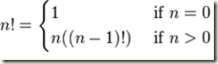## Tuesday, 28 August 2012

### Thinking in recursive way–Mathematics

“I simply forget recursion and always use debuggers to understand it. Debuggers are hated by mathematical programmers. Why you need debuggers btw when you use math to prove your program will work for all corner cases ?”

Introduction

The funny thing about mathematics and programming is that, if you pick one, you will drop another. A perfect example of chicken and egg problem. But programs written for critical applications always goes for mathematical analysis.

To understand Recursion, you need to know some mathematical functions which already aptly follow recursion.

Lets see the factorial function which is defined with below,Lets see an example, where n=4

```4! = 4 x 3!
4! = 4 x 3 x 2!
4! = 4 x 3 x 2 x 1!
4! = 4 x 3 x 2 x 1 x 1```
`Concept`
`   Ok. Factorial is basic. We all knew about all the mathematical functions and their direct mapping with recursion.`
`  Now, where to use recursion? just for mathematical functions? This is the question of programmers, not the mathematicians. :)`
`  I hope you read the previous post on Loop invariant. That is the basis for deciding whether to go for recursion or not. :)`
`  Let’s say, you need to maintain multiple values constant, logged for future processing in a loop. You usually would go for using stacks or some data structure to keep a log of all data required in future. `
`Example:`
`   count the number of nodes in a tree`
`   How to think about this problem loud out? usually people mug up things. :) But that’s not the right way.`
`A simple logic, start with 0 nodes in a tree, means tree variable is NULL.`
`This is base condition. Then, what we need to do, we need count of left tree and right tree.`
`The code goes like below, so simple,`
`if(tree == NULL)`
`  return 0;`
` `
`return count(tree->left) + 1 + count(tree->right);`

Mathematical representation:

count(t) =   0, when t=0

1, when t=1

count(t->left) + count(t->right), when t > 1

Let’s try for 3,

count(3 node tree) = count(1) + count(left + right node)

= 1 + count(1 left node) + count(1 right node) = 3

`Analysis`
`  Here, the loop invariant is at any time, we have the node’s visited on hand. that is, if we have visited all tree->left, we start visiting tree->right. Once we are done with all tree->right, we reach base condition for every node. If the node count is 1, then its 1. :)`
` This is what works out simply for anything that uses recursion, quick sort, merge sort.. any algorithm we see, the base condition value is the important one. After you design the base condition in the form of mathematical function, writing recursive program is simpler.`